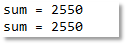# java循环语句之while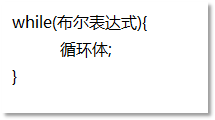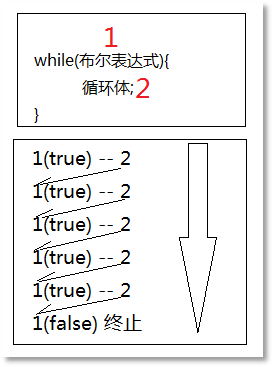``````while(true){
System.out.println("死循环");
}``````

``````int i = 0;
while(i < 10){
i++;
System.out.println("i = " + i);
}``````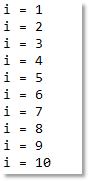``````int j = 1;
while(j <= 10){
System.out.println("j = " + j);
j++;
}``````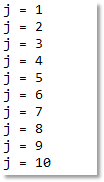``````int i = 0;
while(i < 10){
System.out.println("i = " + (++i));
}``````

``````int j = 1;
while(j <= 10){
System.out.println("j = " + j++);
}``````

``````int sum = 0;
int i = 0;
while(i <= 100){
if(i % 2 == 0){
sum += i;
}
i++;
}
System.out.println("sum = " + sum);

sum = 0;
int j = 0;
while(j <= 100){
sum += j;
j += 2;
}
System.out.println("sum = " + sum);``````